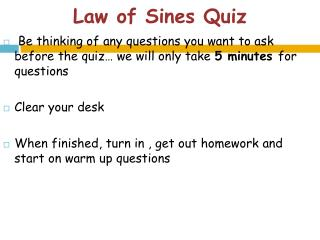# Law of Sines Quiz - PowerPoint PPT PresentationDownload PresentationLaw of Sines Quiz

Law of Sines Quiz
Download Presentation## Law of Sines Quiz

- - - - - - - - - - - - - - - - - - - - - - - - - - - E N D - - - - - - - - - - - - - - - - - - - - - - - - - - -
##### Presentation Transcript

1. Law of Sines Quiz • Be thinking of any questions you want to ask before the quiz… we will only take 5 minutes for questions • Clear your desk • When finished, turn in , get out homework and start on warm up questions

2. Warm Up Mar. 1st • Solve the triangle. • a = 9, b = 3, c = 11 • To approximate the length of a marsh, a surveyor walks 380 meters from point A to B, then turns 80° and walks 240 meters to point C. Approximate the length AC of the marsh. B 80° C A

3. Homework Questions??

4. Area of Triangles

5. B c a C A b Formulas • Area of a triangle = ½(base)(height)

6. Examples • Find the area of a triangular lot having two sides of lengths 90 meters and 52 meters and an included angle of 102°.

7. Examples (cont.) • Find the area of the triangle with the given information. A = 38°45’, b = 67, c = 85

8. Heron’s Area Formula • The Law of Cosines can be used to establish the Heron’s Area Formula, named after the Greek mathematician Heron. • Given any triangle with sides of lengths a, b and c, the area of the triangle is where s is the semiperimeter and s = (a + b + c)/2

9. Examples (cont.) • Find the area of the triangular region having side lengths a = 43, b = 53 and c = 72.

10. Applications • Deer populations in national parks average 14 animals per square kilometer. If a triangular region with sides of 3 km, 4 km and 6km has a population of 50 deer, how close is the population on this land to the average national park population?

11. Examples (cont.) • Use the most appropriate formula to find the area of each triangle. • a = 2, b = 3, c = 4 • a = 2, b = 4, C = 50° • a = 3, b = 4, c = 5

12. Homework Law of Sines & Cosines and Area Practice